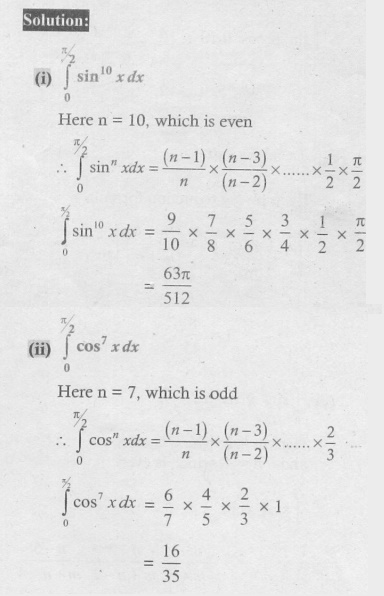Home | | Maths 12th Std | Exercise 9.6: Reduction Formulae

# Exercise 9.6: Reduction Formulae

Maths Book back answers and solution for Exercise questions - Mathematics : Applications of Integration: Reduction Formulae: Exercise Problem Questions with Answer, Solution

EXERCISE 9.6

Evaluate the following: